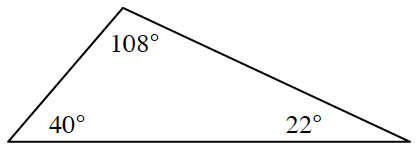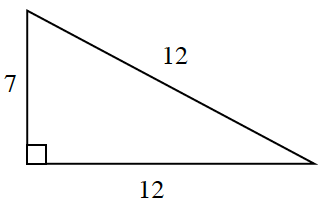### Home > INT3 > Chapter 7 > Lesson 7.2.1 > Problem7-56

7-56.

WHAT’S WRONG WITH THE DIAGRAM?

After drawing some diagrams on his paper, Stephan thinks there is something wrong with his diagram labels. Examine each diagram below and decide whether or not the triangle could exist. If it cannot exist, explain why not.

1.What must be the sum of the angles in a triangle?

Impossible, because the sum of the angle measures is less than $180^\circ$.

1.Look at the length of the hypotenuse and the length of the bottom leg. Is there something odd there?

Impossible, because a leg cannot be congruent to the hypotenuse.